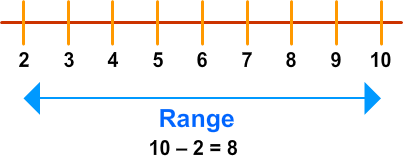# Range

Print Rate 0 stars
Lesson size:
Message preview:
Someone you know has shared lesson with you:

To play this lesson, click on the link below:

https://www.turtlediary.com/lesson/range.html

Hope you have a good experience with this site and recommend to your friends too.

Login to rate activities and track progress.
Login to rate activities and track progress.

The range is the difference between the least and greatest numbers in the set.Example

• Consider the following set: 118, 154, 196, 211, 253.

Find the range.

The least number in the set is 118.

The greatest number is 253.

The range is the difference between these two numbers: 253 - 118 = 135.

So, the range of given set of numbers is 135.

Example

• What is the range of the following set: 15, 0, 8, 23.

The set is not written in order from least to greatest this time.

However, we can still identify the least and greatest numbers in the set without too much trouble.

The least number is 0.

The greatest number is 23.

Find the difference: 23 - 0 = 23

So, the range is 23.

Finding mean, median, mode and range from a chart

A chart is something we use to organize and see numbers better.

Here's a chart that shows 4 children and how many siblings they have:

Child
Siblings
Abby
1
Bob
3
Caitlyn
2
Dan
2
Mean

Add all the number of siblings and divide by the total number of kids.

1 + 3 + 2 + 2 = 8

8 ÷ 4 = 2

So, the mean is 2.

Median

Put the number of siblings in order from least to highest and then find the middle number:

1,2,2,3

Since there are two numbers lying in the middle, we will find their mean:

2 + 2= 4

4 ÷ 2= 2

So, the median is 2.

Mode

To find the mode, look for the number of siblings that comes up most often.

1, 3, 2, 2

Here, 2 comes the most often in this set.

So, the mode is 2.

Range

Find the difference between the least and greatest number of siblings in the set.

3 - 1 = 2

So, the range is 2.

## Range

• The range is the difference between the least and greatest numbers in the set.
• To find the range, just subtract the least value of the data set from the largest value.

## Similar Lessons

Become premium member to get unlimited access.